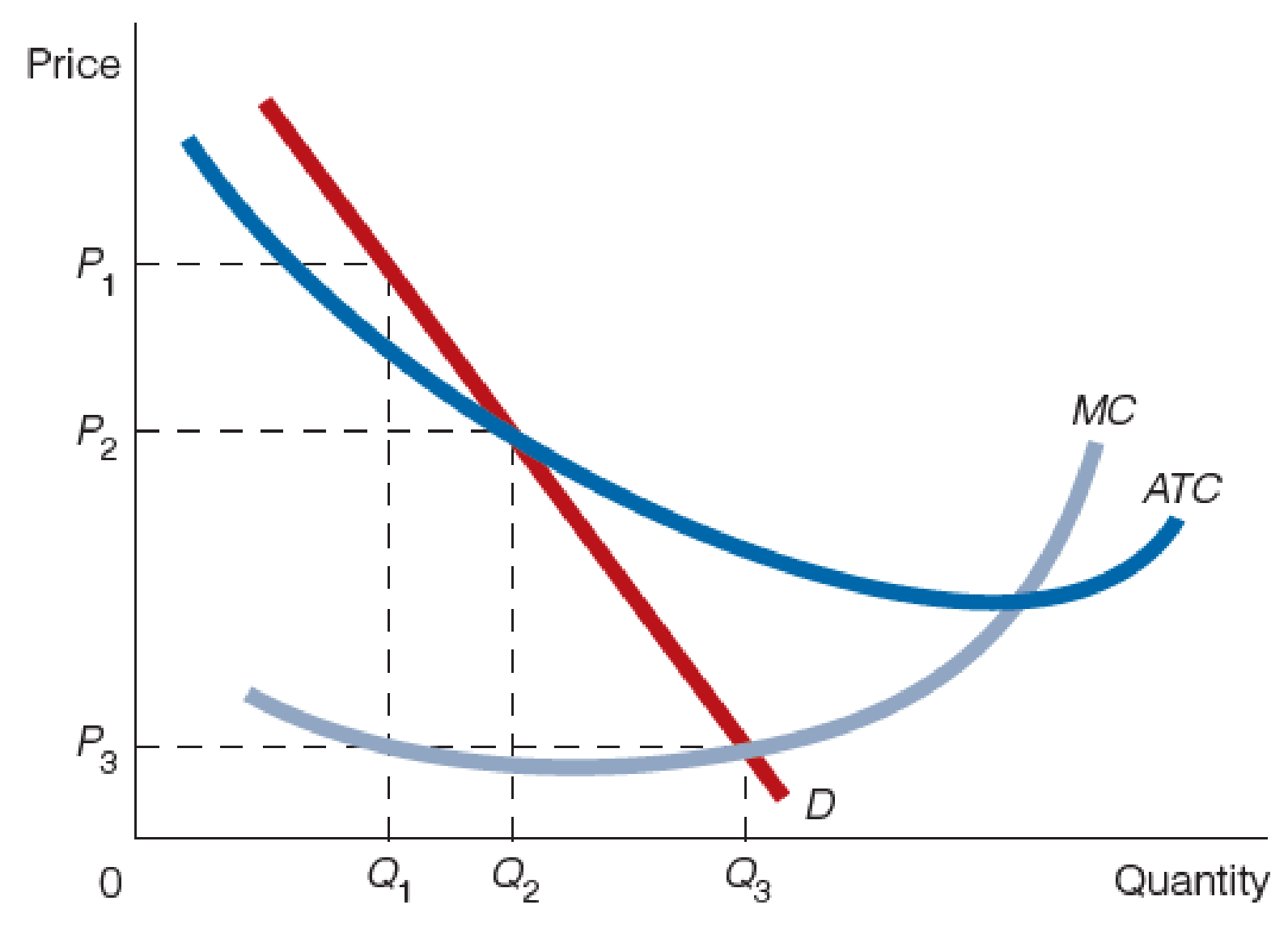Chapter 12, Problem 3WNG

Chapter
Section
Textbook Problem

Use the accompanying figure to answer questions 2–4.3. Will the firm in the figure earn profits if it produces Q3 and charges P3? Explain your answer.

To determine

Determine whether the firm in the figure earns profit, if it produces quantity Q3 and charge price P3.

Explanation

Figure-1 shows the natural monopoly market as follows:

In Figure -1, the horizontal axis measures the quantity and the vertical axis measures the price...

Still sussing out bartleby?

Check out a sample textbook solution.

See a sample solution

The Solution to Your Study Problems

Bartleby provides explanations to thousands of textbook problems written by our experts, many with advanced degrees!

Get Started